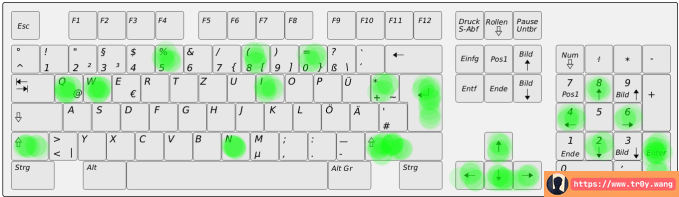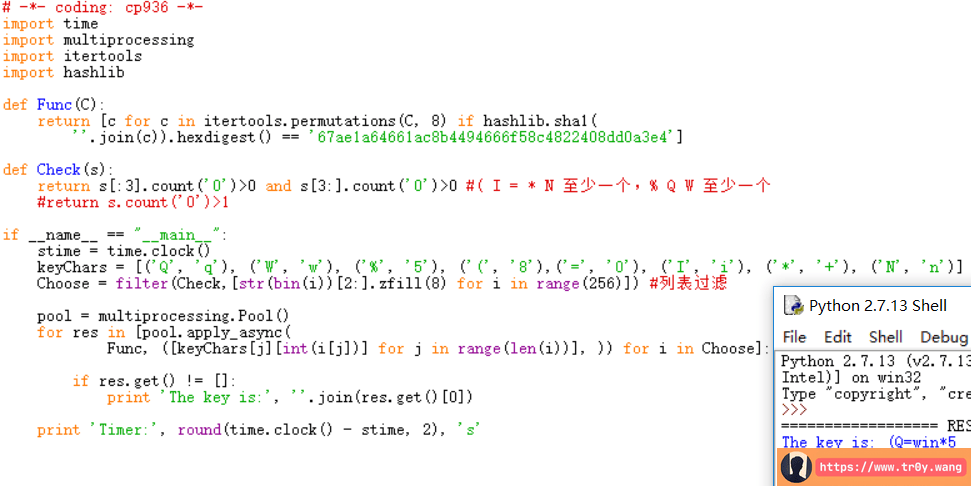Tr0y 10月 11, 2017 15:46:18 本文共 866 字
• 文为知己者书
•• 在其它设备中阅读本文章
•mysterytwisterc3 的一道 LVII 的题

67ae1a64661ac8b4494666f58c4822408dd0a3e4SHA1 作为一种散列函数，是单向不可逆的。通过穷举搜索虽然可以找到明文，但是在空间较大时非常困难。

Q，q，W，w，%，5，8，(，=，0，I，i，*，+，n，N

keyChars = [('Q'， 'q')， ('W'， 'w')， ('%'， '5')， ('8'， '(')，('='， '0')， ('I'， 'i')， ('*'， '+')， ('N'， 'n')]

( I = * N % Q W 这些字符最少出现 2 个

( I = * N 至少出现一个，% Q W 至少出现一个

# -*- coding: cp936 -*-
import time
import multiprocessing
import itertools
import hashlib

def Func(C):
return [c for c in itertools.permutations(C, 8) if hashlib.sha1(
''.join(c)).hexdigest() == '67ae1a64661ac8b4494666f58c4822408dd0a3e4']

def Check(s):
return s[:3].count('0')>0 and s[3:].count('0')>0 #( I = * N 至少一个，% Q W 至少一个
#return s.count('0')>1

if __name__ == "__main__":
stime = time.clock()
keyChars = [('Q', 'q'), ('W', 'w'), ('%', '5'), ('(', '8'),('=', '0'), ('I', 'i'), ('*', '+'), ('N', 'n')]
Choose = filter(Check,[str(bin(i))[2:].zfill(8) for i in range(256)]) #列表过滤

pool = multiprocessing.Pool()
for res in [pool.apply_async(
Func, ([keyChars[j][int(i[j])] for j in range(len(i))], )) for i in Choose]:

if res.get() != []:
print 'The key is:', ''.join(res.get())

print 'Timer:', round(time.clock() - stime, 2), 's'End

What do you think?